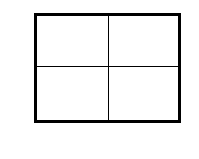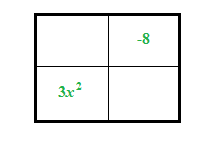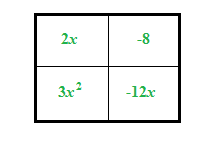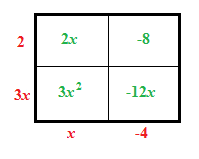### Home > CAAC > Chapter 10 > Lesson 10.4.2 > Problem10-140

10-140.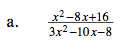Use a generic rectangle to factor the expressions.

3x² −10x + 16
= (3x + 2)(x − 4)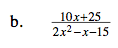Factor the denominator with a generic rectangle. Factor the numerator by finding a common factor.

$\frac{5}{x-3}$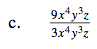Reduce by looking for factors in the numerator and denominator that make one.

$\frac{9}{3}$